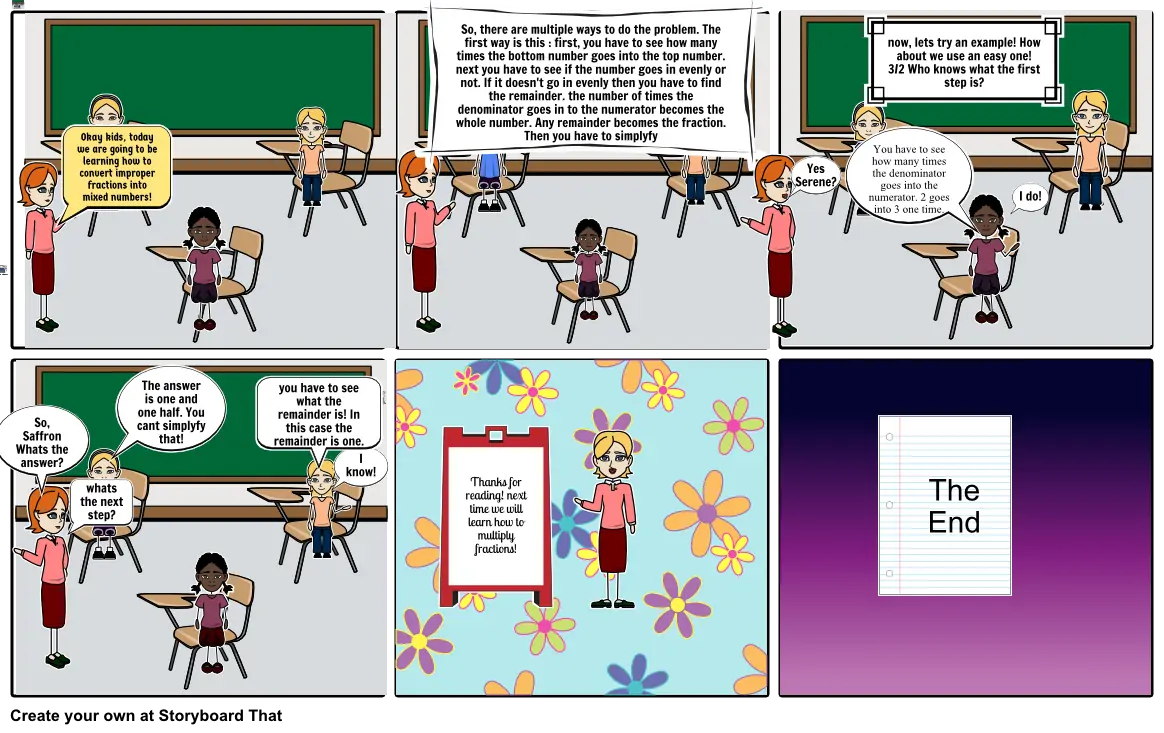# math storyboard#### Storyboard Text

• Okay kids, today we are going to be learning how to convert improper fractions into mixed numbers!
• So, there are multiple ways to do the problem. The first way is this : first, you have to see how many times the bottom number goes into the top number. next you have to see if the number goes in evenly or not. If it doesn't go in evenly then you have to find the remainder. the number of times the denominator goes in to the numerator becomes the whole number. Any remainder becomes the fraction. Then you have to simplyfy
• Yes Serene?
• You have to see how many times the denominator goes into the numerator. 2 goes into 3 one time.
• now, lets try an example! How about we use an easy one! 3/2 Who knows what the first step is?
• I do!
• So, Saffron Whats the answer?
• whats the next step?
• The answer is one and one half. You cant simplyfy that!
• you have to see what the remainder is! In this case the remainder is one.
• I know!
• Thanks for reading! next time we will learn how to multiply fractions!
• The End
##### Over 20 Million Storyboards Created